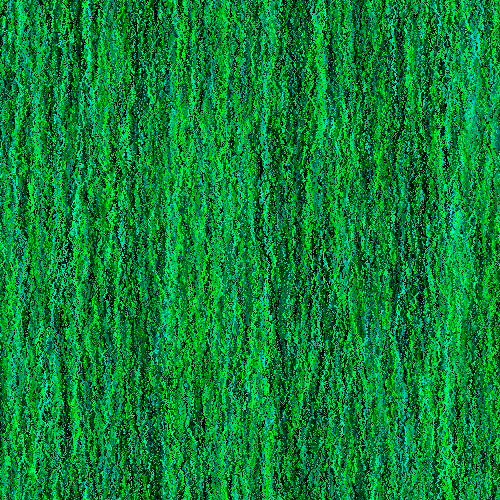# Vine

example processing animation nature generative-art 100-days-of-code
``````float x;
float y;

float g;
float b;

void setup(){
size(600, 300);
x = width/2;
y = height;

g = random(255);
b = random(g);

background(0, 0, random(255));
noSmooth();
}

void draw(){

x += random(-1, 1);
y -= random(1);

if(x < 0){
x = width;
}
if(x > width){
x = 0;
}

if(y < 0){
y = height;
}

g += random(-10, 10);
g = constrain(g, 0, 255);

b += random(-10, 10);
b = constrain(b, 0, g);

stroke(0, g, b);
point(x, y);
}
``````

This code starts out with a point in the bottom middle of the screen. It then moves the point up a little bit, and randomly a little bit to the left or right. It also randomly changes the color to a shade of green. It repeats that process every frame, and the effect looks like a vine growing up the screen. If you leave it running long enough, you get something like this:Code Editor

See the Pen by Happy Coding (@KevinWorkman) on CodePen.

## Tweak Ideas

• Change the color and direction of the vine.
• Randomly draw flowers and leaves on the vine.
• Instead of always crawling up, make it so the vine crawls down sometimes. Or spirals!
• Instead of resetting to the bottom when it goes off the top of the screen, have the vine reappear on a random side. For example, have it randomly reappear on the right side (and have it go left) or the left side (and have it go down) or even back at the top (and have it go down)!